# 3.3.3. Which Plot Type Should I Use?¶

This a brief listing of common graphs and their functions

The functions below are but a little tasting of common plots, and I’m not specifying parameters beyond the utterly necessary. pd and sns functions get their flexibility from the wide assortment of parameters you can alter. Changing the parameters a bit can produce large (and interesting!) alterations. For example, col and hue typically multiply the amount of info in a graph.

You can either read the function’s documentation (and I frequently do!) via SHIFT+TAB or look through the graph example galleries here and here until you see graphs with features you want, and then you can look at how they are made.

Tip

I would absolutely bookmark these links:

## 3.3.3.2. Faceting¶

Facets allow you to present more info on a graph by designing a plot for a subset of the data, and quickly repeating it for other parts.

You can think of facets as either

1. creating subfigures

• the pairplot below creates subfigures for each combination of variables in the dataset

• the Anscombe example makes subfigures for subsets of the data

2. or overlaying figures on top of each other in a single figure

• the categorical boxplot below does this for each sub group

Let’s look at some examples quickly:

import seaborn as sns
import matplotlib.pyplot as plt

iris = sns.load_dataset("iris")
sns.pairplot(iris)
plt.suptitle('Faceting by repeating scatter plots for each pair of variables',fontsize=18)
plt.subplots_adjust(top=0.95) # Reduce plot to make room for the title
plt.show()

# note: .set(title) doesn't work here - it tries to title the individual subfigures (axes)
#       to title the whole thing, I had to use suptitle.

sns.pairplot(iris, hue="species")
plt.suptitle('Faceting by overlaying figures by group',fontsize=18)
plt.subplots_adjust(top=0.95) # Reduce plot to make room for the title
plt.show()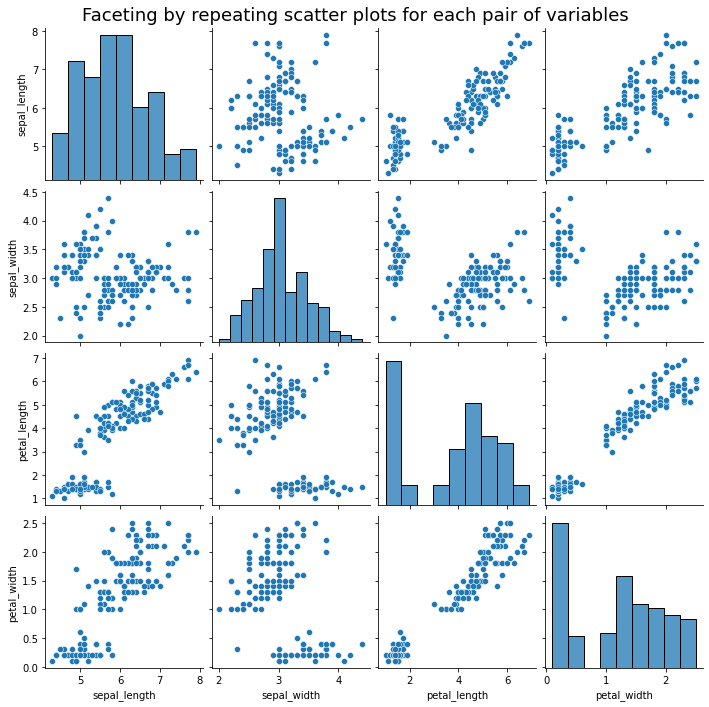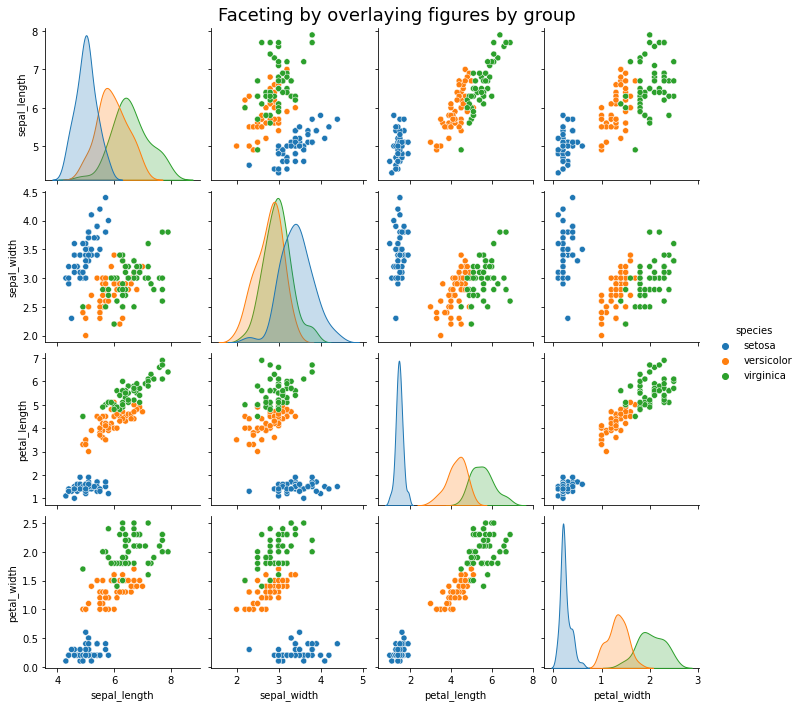Boxplot by group: Just use the x and y arguments together.

sns.boxplot(x="species",y="petal_width", data=iris,)
plt.show()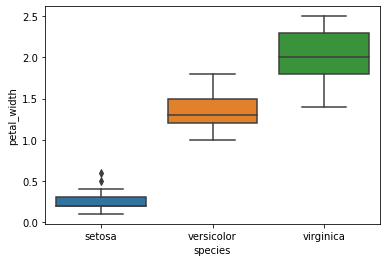An example of faceting via the col argument. Using row instead does what you’d think. Protip: You can use row and col together to make a grid of groups.

sns.lmplot(data=iris,x='petal_width',y="petal_length",col="species")
plt.show()
sns.lmplot(data=iris,x='petal_width',y="petal_length",col="species")
plt.show()

sns.lmplot(data=iris,x='petal_width',y="petal_length",hue="species")
plt.show()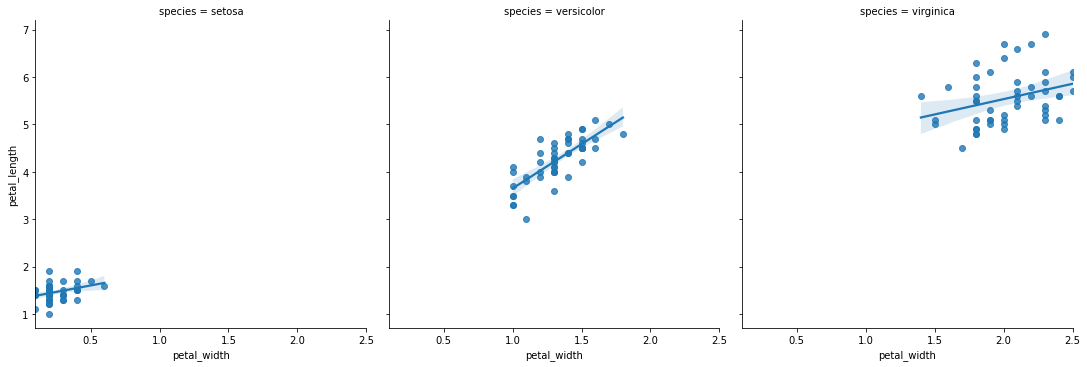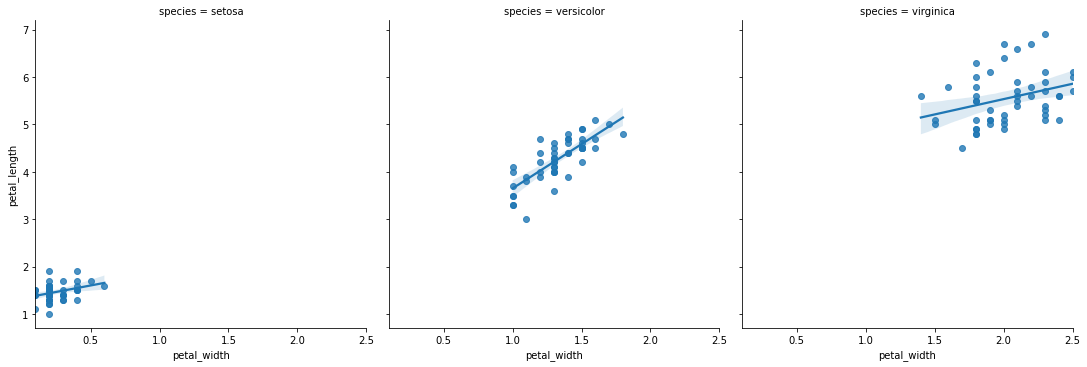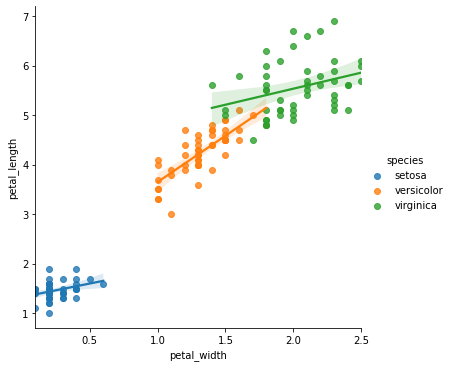### 3.3.3.2.1. I want to Facet my figure, but…¶

Problem: The variable you want to facet/group by is

• (A) continuous variable

• or (B) a variable with too many values.

Solutions:

• (A) - partition/slice/factor your variable into bins using panda’s cut function.

• (B) - re-factor the variables into a smaller number of groups, or only graph some of them.

For example: Say you want to plot how age and death are related, and you want to plot this for healthy people and less-healthy people. So you collect the BMI of individuals in your sample. Let’s say that BMI can take 25 values from 15 to 40. The problem is plotting 20 sub-figures is probably excessive. The solution is to use the cut function to create a new variable which is four bins of BMI according to the UK’s NHS: underweight (BMI<18.5), healthy (BMI 18.5-24.5), overweight (BMI 24.5-30), obese (BMI>30).

## 3.3.3.3. Practice: Thinking and planning¶

Questions: Which type of graph (bar, line, or histogram) would you use?

1. The volume of apples picked at an orchard based on the type of apple (Granny Smith, Fuji, etcetera).

2. The number of points for each game in a basketball season for a team.

3. The count of apartment buildings in Chicago by the number of individual units.

Answers